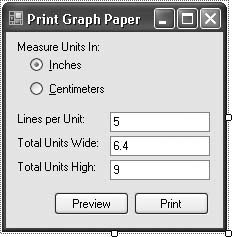# Recipe 11.8. Creating Graph Paper

#### Problem

You've run out of graph paper, but you need a sheet of it right now. You'd like to brush up on your .NET printing skills at the same time.

#### Solution

Sample code folder: Chapter 11\GraphPaper

Build a simple application that prints some graph paper for you, using the printing features presented throughout this chapter and various GDI+ methods.

#### Discussion

Create a new Windows Forms project, and add the following controls to the form:

• A RadioButton control named UseInches. Set its Text property to &Inches and its Checked property to true.

• A RadioButton control named UseCentimeters. Set its Text property to &Centimeters.

• A TextBox control named LinesPerUnit.

• A TextBox control named UnitsWide.

• A TextBox control named UnitsHigh.

• A Button control named ShowPreview. Set its Text property to Preview.

• A Button control named SendToPrinter. Set its Text property to Print.

Add informational labels if desired. The form should look something like the one in Figure 11-6.

##### Figure 11-6. User interface for the Graph Paper applicationAdd the following source code to the form's class template:

` Imports System.Drawing.Printing Public Class Form1    Private WithEvents GraphPaper As Printing.PrintDocument    Private Sub ShowPreview_Click( _          ByVal sender As System.Object, _          ByVal e As System.EventArgs) _            Handles ShowPreview.Click       ' ----- Preview the   graph paper.       Dim previewMode As New PrintPreviewDialog       GraphPaper = New Printing.PrintDocument       previewMode.Document = GraphPaper       previewMode.ShowDialog()       GraphPaper = Nothing    End Sub    Private Sub SendToPrinter_Click( _          ByVal sender As System.Object, _          ByVal e As System.EventArgs) _          Handles SendToPrinter.Click       ' ----- Preview the   graph paper.       Dim pageSetup As New PageSetupDialog       GraphPaper = New Printing.PrintDocument       pageSetup.Document = GraphPaper       If (pageSetup.ShowDialog( ) = _          Windows.Forms.DialogResult.OK) Then _          GraphPaper.Print()       GraphPaper = Nothing    End Sub    Private Sub GraphPaper_PrintPage(ByVal sender As Object, _          ByVal e As Printing.PrintPageEventArgs) _          Handles GraphPaper.PrintPage       ' ----- Printing of the graph paper occurs here.       Dim unitLines As Single = CSng(LinesPerUnit.Text)       Dim totalWidth As Single = CSng(UnitsWide.Text)       Dim totalHeight As Single = CSng(UnitsHigh.Text)       Dim x1, y1, x2, y2 As Single       Dim fineBlackPen As New Pen(Color.Black, 0.00001)       Dim eachLine As Integer       Dim factor As Single       ' ----- Set the units.       If (UseInches.Checked = True) Then          e.Graphics.PageUnit = GraphicsUnit.Inch          factor = 1.0!       Else          e.Graphics.PageUnit = GraphicsUnit.Millimeter          factor = 10.0!       End If       ' ----- Draw the vertical lines.       For eachLine = 0 To CInt(totalWidth * unitLines)          x1 = factor + (eachLine * factor) / unitLines          y1 = factor          x2 = x1          y2 = y1 + (totalHeight * factor)          If ((eachLine Mod unitLines) = 0) Then             ' ----- Each unit marker is thicker.             fineBlackPen.Width = 0.01 * factor          Else             fineBlackPen.Width = 0.000001 * factor          End If          e.Graphics.DrawLine(fineBlackPen, x1, y1, x2, y2)       Next eachLine       ' ----- Draw the horizontal lines.       For eachLine = 0 To CInt(totalHeight * unitLines)          x1 = factor          y1 = factor + (eachLine * factor) / unitLines          x2 = x1 + (totalWidth * factor)          y2 = y1          If ((eachLine Mod unitLines) = 0) Then             ' ----- Each unit marker is thicker.             fineBlackPen.Width = 0.01 * factor          Else             fineBlackPen.Width = 0.000001 * factor          End If          e.Graphics.DrawLine(fineBlackPen, x1, y1, x2, y2)       Next eachLine       ' ----- Limit output to a single page.       e.HasMorePages = False    End Sub End Class `

This program builds on the recipes presented throughout this chapter. It creates distinct PrintDocument (with WithEvents specified), PrintPreviewDialog, and PageSetupDialog classes, and it responds to the print document's PrintPage event to perform the actual printing.

The code simply loops through the specified number of vertical and horizontal lines destined for the output based on the user's input, and draws lines at each interval position. The e.Graphics.PageUnit property lets the code easily process both English and metric measurement systems, although the lack of a basic centimeter unit requires the code to combine the millimeter unit with a scaling factor.Visual Basic 2005 Cookbook: Solutions for VB 2005 Programmers (Cookbooks (OReilly))
ISBN: 0596101775
EAN: 2147483647
Year: 2006
Pages: 400

Similar book on Amazon